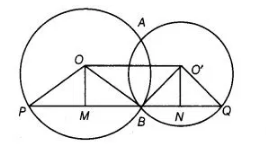# Two circles with centres O and O’ intersect`
Question:

Two circles with centres O and O’ intersect at two points A and B. A line PQ is drawn parallel to OO’ through A (or B) intersecting the circles at P and Q.

Prove that PQ =2 OO’.

Solution:

Given, draw two circles having centres O and O’ intersect at points A and 8.Also, draw line $P Q$ parallel to $O O^{\prime}$.

Construction Join $O O^{\prime}, O P, O^{\prime} Q, O M$ and $O^{\prime} N$.

To prove $P Q=200^{\prime}$

Proof in $\Delta O P B$, $B M=M P$ [OM is the perpendicular bisector of $P B$ ]

and in $\triangle O^{\prime} B Q$, $B N=N Q$ $\left[O^{\prime} N\right.$ is the perpendicular bisector of $\left.B Q\right]$

$\therefore$ $B M+B N=P M+N Q$

$\Rightarrow \quad 2(B M+B N)=B M+B N+P M+N Q$

[adding both sides $B M+B N$ ]

$\Rightarrow$ $2 O O^{\prime}=(B M+M P)+(B N+N Q)$

$\left[\because O O^{\prime}=M N=M B+B N\right]$

$=B P+B Q=P Q$

$\Rightarrow \quad 200^{\prime}=P Q$

Hence proved.